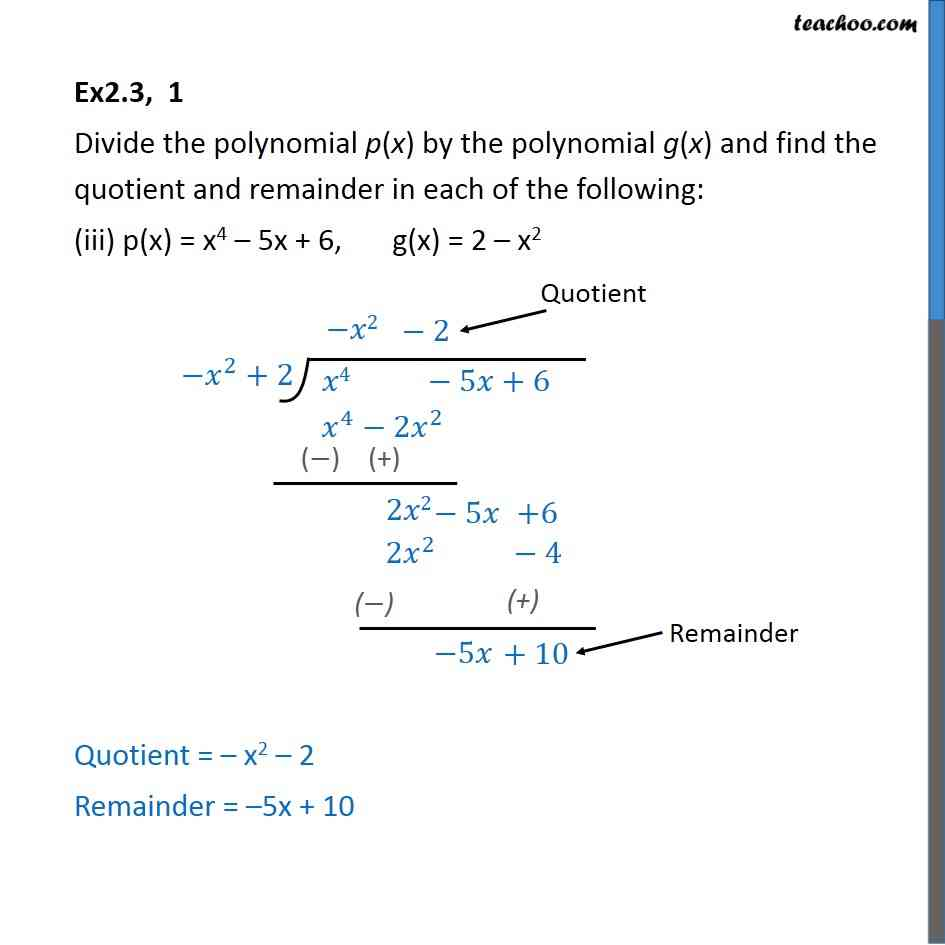Division Algorithm

Chapter 2 Class 10 Polynomials
Serial order wiseLearn in your speed, with individual attention - Teachoo Maths 1-on-1 Class

### Transcript

Ex2.3, 1 Divide the polynomial p(x) by the polynomial g(x) and find the quotient and remainder in each of the following: (iii) p(x) = x4 – 5x + 6, g(x) = 2 – x2 Quotient = – x2 – 2 Remainder = –5x + 10